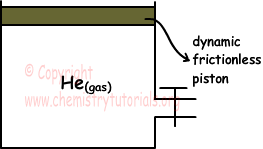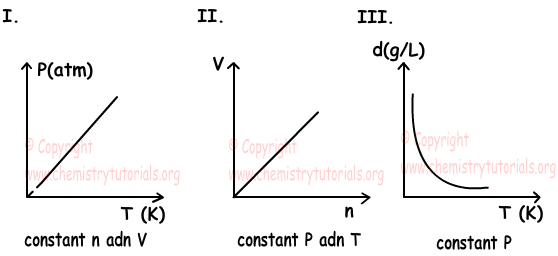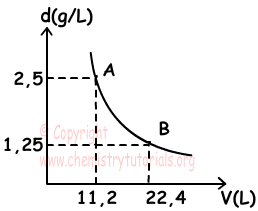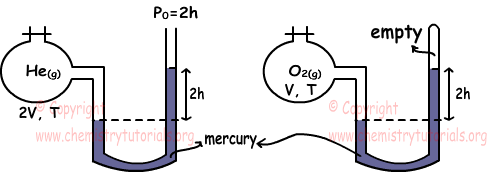EN | ES | DE | NL | RU

## Density of Gases with Examples

Density of Gases with Examples

Density of gases is too small with respect to solid and gas phases. We can find density with following formula;

d(gas)=m(gas)/V(gas)

if we substitute it into the ideal gas law;

P.V=n.R.T  where n=mass/molar mass

P.V=(m/Mm).R.T

P.Mm=(m/V).R.T

P.Mm=d.R.T

d=(P.Mm)/(R.T)

As you can see from the formula; density of gases is directly proportional to pressure and molar mass and inversely proportional to temperature.

Example: Find density of C4H8 at 273 0C and under 2 atm pressure. (H=1, C=12)

Solution: we make unit conventions first;

T=273+273=546 K

P=2 atm

C4H8=4.12+8.1=56 g/mol

Using formula given above;

P.Mm=d.R.T

2.56=d.(22,4/273).546

d=2,5 g/L

Example: If we add some CH4 to container given below under constant temperature; which ones of the following statements are true related to gases in this container? (He=4, C=12, H=1)I. Density of mixture increases

II. Volume increases

III. Pressure increases

Solution:

Molar mass of CH4=12+4.1=16

Since piston of  container is dynamic, when we add CH4, volume of mixture increases. Molar mass of CH4 is greater than He, thus density of mixture also increases.

P.Mm=d.R.T

Increasing in the volume of gas balance pressure and it stays constant.

Example: Which ones of the graphs are true for ideal gas.Solution:

I. Using ideal gas law;

P.V=n.R.T

P=n.R.T/V

Since R, n and V are constant, P is directly proportional to temperature. Graph I is true.

II. Molar volume is V/n. Using ideal gas law;

V/n=R.T/P

Since R, P and T are constant V/n must be constant. Thus second graph is false, line showing relation between V and n must be parallel to n.

III. We write ideal gas law for density;

d=P.Mm/R.T

Mm, R and P are constant , thus d is inversely proportional to T. III. graph is true.

Example: Graph given below shows density vs. volume relation of X(gas) at 00C. If the pressure of X(gas) at point A is 1 atm, which ones of the following statement are true for this gas.I. n=1mol

II. Pressure at point B is 0,5 atm

III. Molar mass of gas is 56 g

Solution:

I. Ideal gas law at point A;

PA.VA=n.R.T

T=0 0C or 273 K

V=11,2 Liters

P=1 atm

n=PA.VA/R.T=(1.11,2)/(22,4/273).273)=0,5mol

I is false.

II. n and T are constant, thus we can write;

PA.VA=PB.VB

(1.11,2)=PB.22,4

PB=0,5 atm, II is true

III. density at point A is;

dA=PA.Mm/R.T

Mm=(dA.R.T)/PA=(2,5.(22,4/273).273)/1

Mm=56 g/mol

1 mol gas contains 56 g, so III is true.

Example: Which ones of the following statements are true for He and O2 gases under same temperature. (He=4, O=16)I. nHe=4nO2

II. dHe=8dO2

III. Average kinetic energies of He and O2 are equal.

Solution:

We find pressures of gases using manometers.

PHe=2h+2h=4h

PO2=2h

To find relation between number of moles of gases we use ideal gas law.

PHe.VHe=nHe.R.THe

nHe=4h.2V/R.T

PO2.VO2=nO2.R.TO2

nO2=2h.V/R.T

Ratio of nHe and nO2

nHe/nO2=4/1 Thus, I is true.

We find density of gases again using ideal gas law.

Molar mass of He=4 and Molar mass of O2=2.16=32

dHe=PHe.MHe/R.T

dHe=4h.4/R.T

dO2=PO2.MO2/R.T

dO2=2h.32/R.T

Ratio de las densidades;
dHe/d/O2=1/4 so, II is false.

III. Since temperature of gases same, their average kinetic energies are also same. III is true.

Gases Exams and  Problem Solutions

The Original Author:

© Copyright www.ChemistryTutorials.org, Reproduction in electronic and written form is expressly forbidden without written permission of www.ChemistryTutorials.org. Privacy Policy